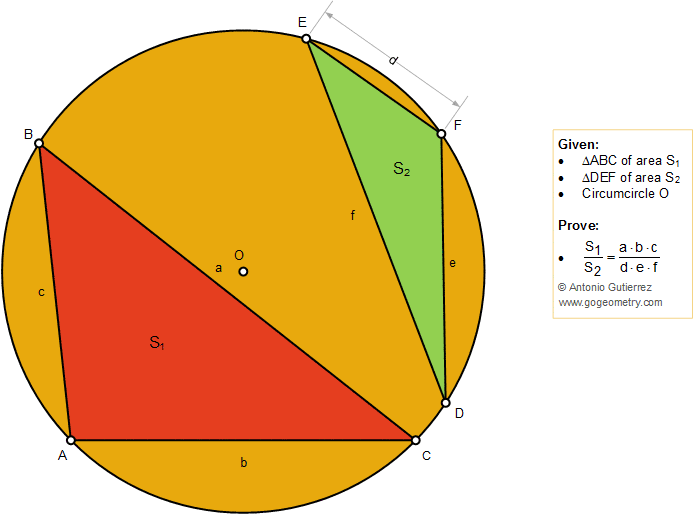# Geometry Problem 1391: Two Triangles, Ratio of Areas, Circumcircle

### Proposition

The figure below shows two triangles ABC and DEF with areas S1 and S2 inscribed in a circle O. Prove that S1/S2 = (a.b.c)/(d.e.f)